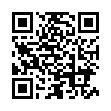# Lab 5 Titrations Fall 2015(1) .pdf

### File information

Author: Tanner

This PDF 1.5 document has been generated by MicrosoftÂ® Word 2010, and has been sent on pdf-archive.com on 11/10/2017 at 20:16, from IP address 104.194.x.x. The current document download page has been viewed 587 times.
File size: 616 KB (13 pages).
Privacy: public file

### Document preview

Experiment 5:
Acid–Base Titrations
Safety First!
Safety goggles must be worn at all times in the laboratory!
Potential Hazards

HCl (hydrochloric acid) is corrosive, can cause painful chemical burns, and will eat holes in your
clothes. Rinse hands with water if you get any on you. See your TA for help cleaning up a spill
immediately.
NaOH (sodium hydroxide) is caustic and can cause chemical burns. These are not painful at first,
so you may not realize if you have gotten it on your skin; wash hands after using.
Indicator solutions are potentially flammable and toxic; keep them away from open flames and do
not ingest them.

Waste Disposal
 All waste containing acid and base must be disposed of in the hazardous-waste container.

Experiment Objective(s):
Standardize a solution of base using the analytical technique known as titration.
Measure the quantity of stomach acid that can be neutralized by antacid tablets.

Learning Objectives:
In this experiment you will learn to perform a titration, select suitable indicator, detect an
endpoint, and determine the amount of active ingredient in the antacid tablet.

Background:
In preparation for this experiment you should review chapter 4 ,sections 4.5 and 4.7 of your
textbook, Chemistry (Chang &amp; Goldsby), Custom Edition for IIT, 2015.
You should also study the technique(s) of titration posted in the Techniques Folder of
Blackboard.
Standardization of a Sodium Hydroxide Solution
Titration is the quantitative analysis of the contents of a solution. The solution under study is
called the analyte or titrand. The solution that is used to determine the contents of the analyte is
called the titrant. In this reaction, the titrant is sodium hydroxide solution, NaOH (aq). For a
proper acid-base titration, it is essential to know the concentration of the NaOH (aq) that is
required to neutralize the acidic analyte. This concentration can be calculated by accurately
weighing a solid sample of sodium hydroxide and dissolving in water to form a known volume
of solution. However, it is difficult to accurately weigh sodium hydroxide because it is
hygroscopic (meaning that it absorbs water vapor readily from air) forming a partially hydrated
solid. Therefore, a solution of NaOH (aq) is usually standardized using an acid known as a

Page 1

Experiment 5:
Acid–Base Titrations
primary standard. A primary standard must be a solid, stable compound that is not hygroscopic,
can be easily handled, and is available in very pure form.
For this experiment, you will standardize a solution of NaOH (aq), which has an approximate
concentration of 0.3 M, by using potassium hydrogen phthalate, KHP, as a primary standard.
KHP has a chemical formula KHC8H4O4 and a molecular weight of 204.23 g/mole. When, KHP
is dissolved in water to form an aqueous solution, the potassium cation dissociates from the
hydrogen phthalate anion according the equation:
+

-

KHC8H4O4 (s) → K (aq) + HC8H4O4 (aq)
The hydrogen phthalate anion has one acidic proton, which reacts stoichiometrically with one
OH– according to the following equation:

2-

OH– (aq) + HC8H4O4 (aq) → C8H4O4 (aq) + H2O (l)
The previous equation is often written in abbreviated form as

2-

OH– (aq) + HP (aq) → P (aq) + H2O (l)
where the letter “P” stands for “phthalate”.
For the highest accuracy, a sample size is chosen such that it will consume as large a volume of
the base as possible without exceeding the capacity of the buret. At the endpoint, the number of
moles of NaOH equals the number of moles of KHP. For example, if a 25 mL buret is used, an
amount of KHP is chosen such that it will require approximately 20 mL of 0.3 M NaOH solution
to reach the endpoint. Thus, about 0.006 moles, or 1.2 g, of KHP is needed. Keep in mind that
you will be using a 50 mL buret in your experiment.
M (NaOH) = M (KHP) =

moles (KHP)
V (NaOH) in liters

=

g (KHP)
204.23 g/mole

×

1000 𝑚𝐿/𝐿
𝑚𝐿 (𝑁𝑎𝑂𝐻)

Once the NaOH solution has been standardized, it can be used to determine the acid content of
an analyte.
Determination of the Acid Neutralizing Capacity of an Antacid Tablet

The parietal cells secrete hydrochloric acid, HCl (aq) at a concentration of roughly 0.16 M in the
stomach. The flow of HCl (aq) increases when food enters the stomach. If you eat or drink too
much, you may develop heartburn or indigestion. Antacids, such as Tums, are used to neutralize
this excess acid. The active ingredient in Tums is calcium carbonate, CaCO3, a base. There are
Page 2

Experiment 5:
Acid–Base Titrations
also other ingredients, such as binders present in each tablet. On average, a 1.3 gram tablet
contains 0.5 g of calcium carbonate and the rest of the mass consists of inactive ingredients.
HCl (aq) is neutralized by calcium carbonate as illustrated below:
-

CaCO3 (s) + H+ (aq)→ Ca2+ (aq) + HCO3 (aq)
-

HCO3 (aq) + H+ (aq)→ H2CO3 (aq)
H2CO3 (aq)→ CO2 (g) + H2O (l)
To determine the ability of Tums to neutralize acid, we are first going to dissolve the tablet in an
excess amount of acid of known concentration. Some of the HCl (aq) will be neutralized by the
carbonate and some will remain resulting in an acidic solution. We will then perform a titration
with previously standardized NaOH (aq) to determine the amount of excess acid. By knowing
the original amount of HCl (aq) added we will subtract the amount remaining to calculate the
amount of acid that was neutralized by the Tums tablet and therefore we will know the amount
of CaCO3 in the Tums. This method of analysis is called back-titration, where we determine the
amount of base by acidifying it with a known quantity of acid and then titrate it back to neutral.
The reactions above are reversible, which means that carbon dioxide gas, CO2 (g), dissolved in
water will produce some carbonic acid, H2CO3 (aq). This acid will react with the NaOH (aq)
titrant causing inaccurate results. To avoid this problem, it is important to boil the solution when
the carbonate reacts with acid which will remove the carbon dioxide as a gas.
The neutralization reaction of a strong acid, HCl (aq), with a strong base, NaOH (aq), is
HCl (aq) + NaOH (aq)→ NaCl (aq) + H2O (l)
When a solution of hydrochloric acid is exactly neutralized with a solution of sodium hydroxide,
the number of moles of NaOH consumed will equal to the number of moles of HCl initially
present in the analyte. This exact neutralization occurs in a reaction between a strong acid and a
strong base at the “equivalence” point; the point at which equivalent amounts of acid and base
have been added to the reaction. At this point, the following relationship therefore holds true:
(MNaOH)(VNaOH in liters) = (MHCl)(VHCl in liters)
Where M is the concentration in molarity and V is the volume. If three of the above quantities
are known, the fourth can be easily calculated.
Page 3

Experiment 5:
Acid–Base Titrations
Indicator Choice

The pH is a scale used to measure acidity and will be discussed in detail next semester. For
current experiment, it is only necessary to know that neutral solutions have a pH equal to 7,
acidic solutions have pH lower than 7 and basic solutions have a pH greater than 7.
To determine when a solution has been exactly neutralized, an acid-base indicator is used that
changes color in a certain pH range. This color change is termed the “endpoint” of the titration;
the point at which the titration is determined to be complete. For an accurate titration, the
endpoint and the equivalence point must as close as possible. The neutralized solution in our
experiment will have a pH equal to 7 and therefore an indicator that changes color near this pH
should be used for a strong acid-base titration.
To determine the best indicator for the titration, you are given a chart of various indicators with
pH ranges to choose from. Please see below

Page 4

Experiment 5:
Acid–Base Titrations
Procedures:
Equipment and Chemicals

50mL Buret
Ring stand
Clamp
Glass stirring rod
Wash bottle of DI water
Antacid tablets

0.3M NaOH (approx.)
0.3M HCl (approx.)
KHP
Indicator solutions
Hot plate
Paraffin film

Follow the procedures outlined on the following pages; record data, observations, and any
deviations from the prescribed procedure as you work; use blue or black ink. You must have the
“landscape pages” with your data and observations signed by your TA before you leave the lab,
and you will submit them with your lab report. Be sure to always record the units and the correct
number of significant figures for any measured value.

Page 5

Experiment 5:
Acid–Base Titrations
Pre-lab Questions (10pts)
Pre-lab questions must be completed and turned in at the beginning of the lab period. If you
have not completed the pre-lab questions, you will not be allowed to complete the lab.
1. When the hydrochloric acid is added to the calcium carbonate, will there be an excess of
HCl(aq) or CaCO3(aq) or will there be stoichiometric equivalents of the two? Show your
calculations.
(4pts)

2. In this lab, you will use a 50mL buret and ~0.3M NaOH (aq). Calculate the mass of
potassium hydrogen phthalate, KHP, needed for the standardization of NaOH (aq) in Part B.
Show your calculations. Copy this number into the Procedure Section B.
(3 pts)

3. Which indicator would you choose to perform titration in lab and why? What color change do
you expect to see?
(3pts)

Page 6

Experiment 5
Procedure
SAFETY: Safety goggles are required in lab. HCl
(hydrochloric acid) is corrosive, can cause chemical burns. NaOH
(sodium hydroxide) is caustic and can cause chemical burns.
Indicator solutions are potentially flammable and toxic; keep them
away from open flames and do not ingest them.

Name___________________

Partner(s)_________________________

Data and Observations

Why are you boiling the solution in Step 6?

Note – Ensure tip of burette is not chipped or broken and that
valve turns easily and smoothly.

A. Preparation of Acidified Antacid Tablet Solution
1. Obtain two Tums antacid tablets to prepare solutions for
two individual trials.
2. Weigh each antacid tablet on a weighing paper on the
analytical balance to the nearest milligram. Record the
mass.
3. Transfer the tablets to two clean 250mL Erlenmeyer flasks;
4. Obtain HCl solution of approximately 0.300M
concentration and recording the precise concentration.
This value should be written on the label of the bottle.
6. Heat the flask to boiling on a hot plate. Gently boil the
solution for about 5 min. While heating, use a glass
stirring rod, if necessary, to help break up and disperse the
tablets being careful keep all of the solid in its flask.
7. Set the solutions aside to allow them to cool to room
temperature for the back-titration in Part C.

TA Signature:___________________

Titration 1

Titration 2

Mass of tablet
Concentration of HCl(aq)
Volume of HCl(aq)

How could you improve the precision of the experiment?

Page 7

Experiment 5

Name___________________

Partner(s)_________________________

B. Standardization of NaOH Solution
1. Accurately and precisely weigh out two (2) samples an
appropriate amount of KHP (determined in pre-lab
question 2) and record the values of each.
2. Dissolve each KHP sample in about 50 mL of water. This
process may take a few minutes. Cover two of the
solutions with paraffin film to stop them from reacting
with the air and set them aside until you are to titrate them.
3. Add 2-3 drops of phenolphthalein indicator.
4. Begin adding the sodium hydroxide solution from the buret
while continuously swirling the flask contents. A pink
color will appear at the point where the NaOH mixes with
the flask contents and will quickly disappear with swirling.
5. As the endpoint nears, this color will persist longer. At this
point, do not open the stopcock completely.
6. Placing a white piece of paper under the flask will aid in
observing the color change. When the color persists for 30
seconds after swirling, the endpoint has been reached.
7. Record the final buret reading to the appropriate number of
significant figures.
8. Calculate the concentration in molarity of the NaOH
solution (see calculations section).
9. Refill the buret so that you do not run out of NaOH
solution in the middle of the next titration and repeat steps
3-8, two more times.
10. If the two determinations of concentration differ by more
than 0.002 M, make a fourth measurement.
11. Use an average of these molarities for analyzing the
antacid in the next part of the experiment. Your base
solution is now standardized!

TA Signature:___________________

Why do you need to accurately measure the mass of KHP that you
estimated in the pre-lab assignment?

Approximate mass of KHP need for standardization

Trial 1

Trial 2

Trial 3
(if needed)

Mass of KHP
Concentraion of
NaOH solution
(calculated)

If your buret runs too low on NaOH, how can you continue the trial without
having to start all over?

Page 8

Experiment 5

Name___________________

Partner(s)_________________________

C. Back-titration of the Antacid Tablets
1. Refill the 50mL buret with the now standardized NaOH
and record the initial buret reading.
2. Add 2-3 drops of the indicator you have been assigned to
use to the acidified and cooled antacid tablet solutions.
3. Titrate by adding the sodium hydroxide solution from the
buret while continuously swirling the flask contents.
4. Look for signs of an indicator change associated with your
indicator. As the endpoint nears, this color will persist
longer. At this point, you need to add NaOH solution more
slowly.
5. When the color persists for 30 seconds after swirling, the
endpoint has been reached. Record the final buret reading
to the appropriate number of significant figures.
6. Repeat steps 1-5 for the second sample.

Also,
 Record the mass of active ingredient per tablet from the
manufacturer’s label.

Titration 1

Titration 2

Manufacturer’s data
Mass of active ingredient,
CaCO3

TA Signature:___________________

Page 9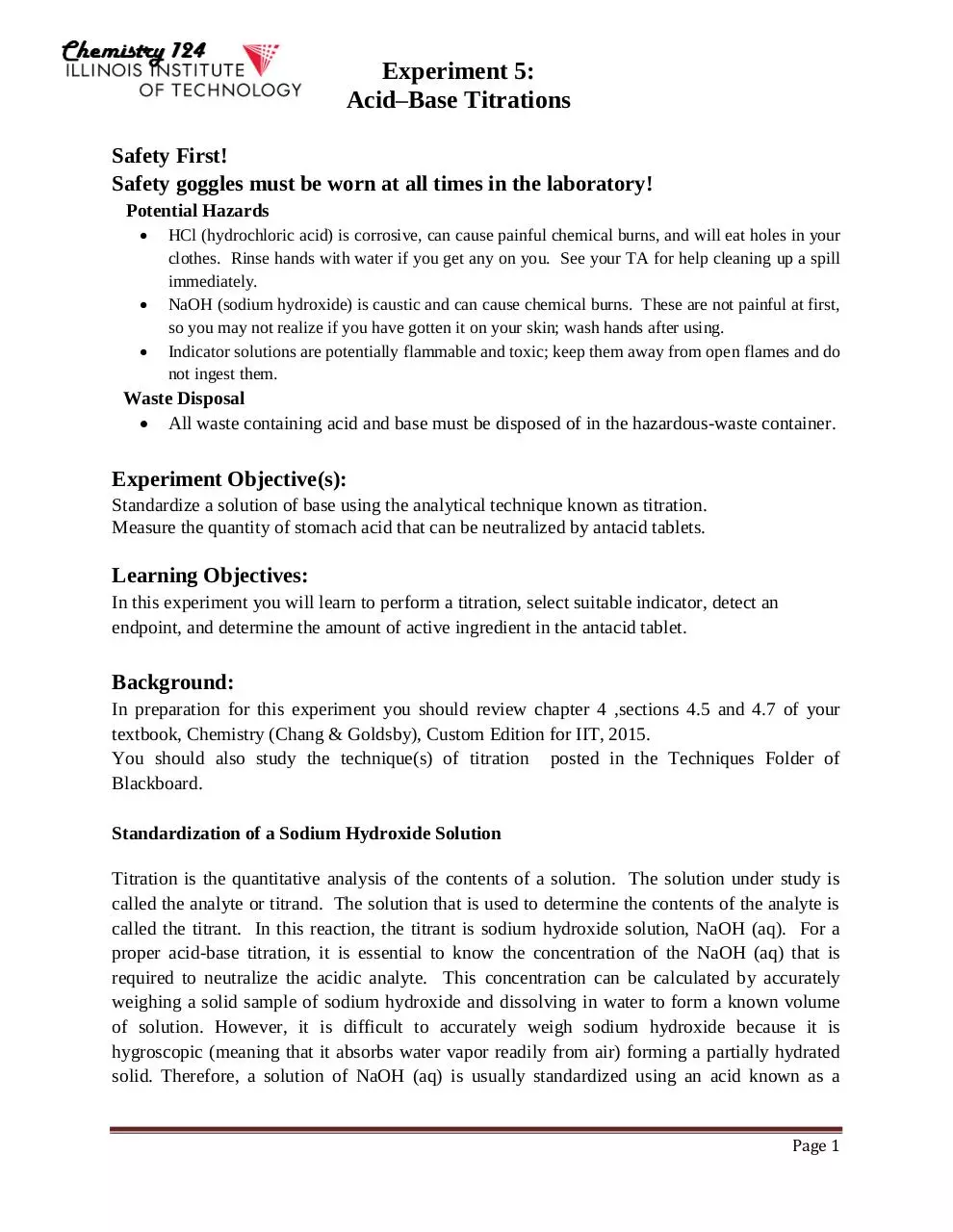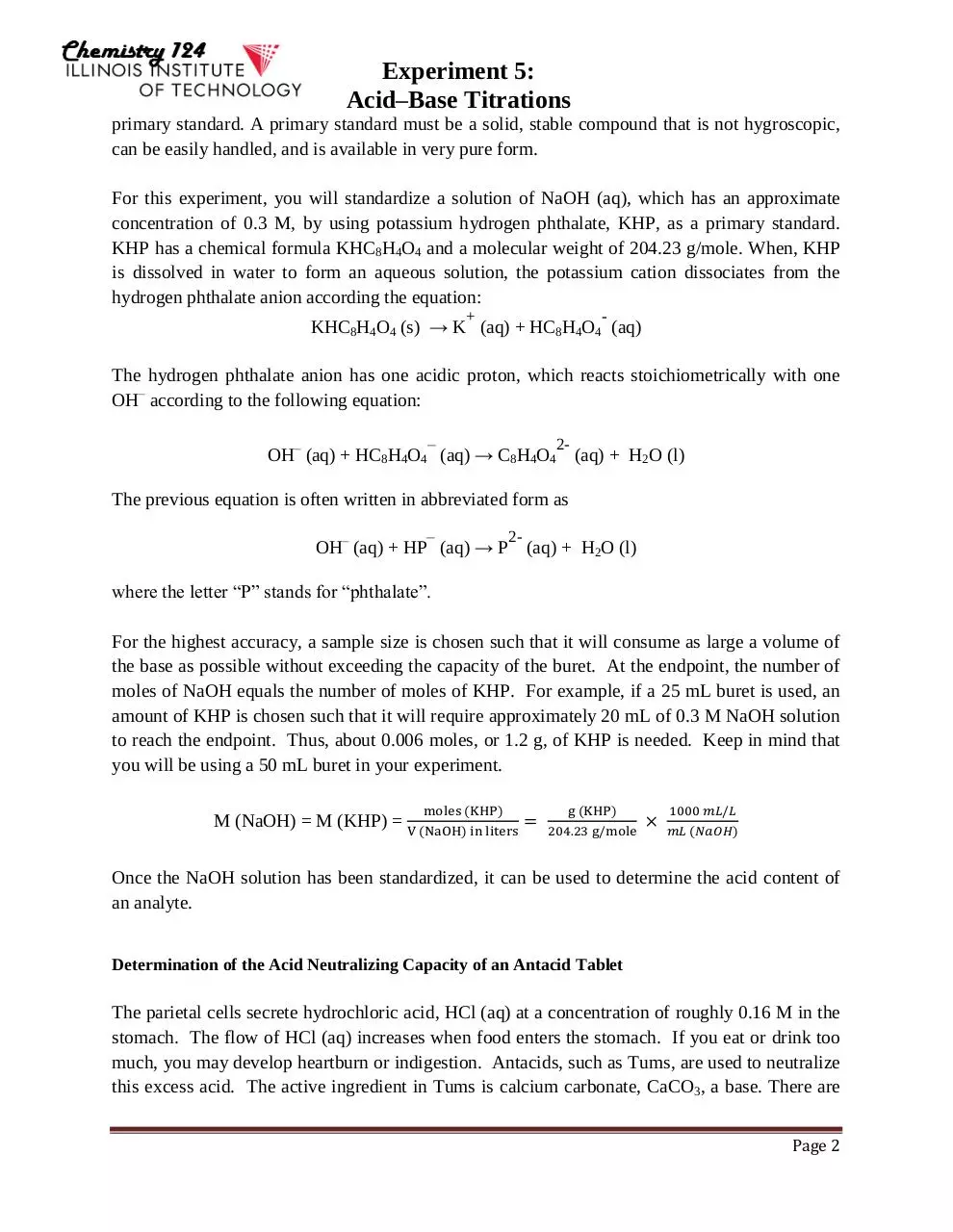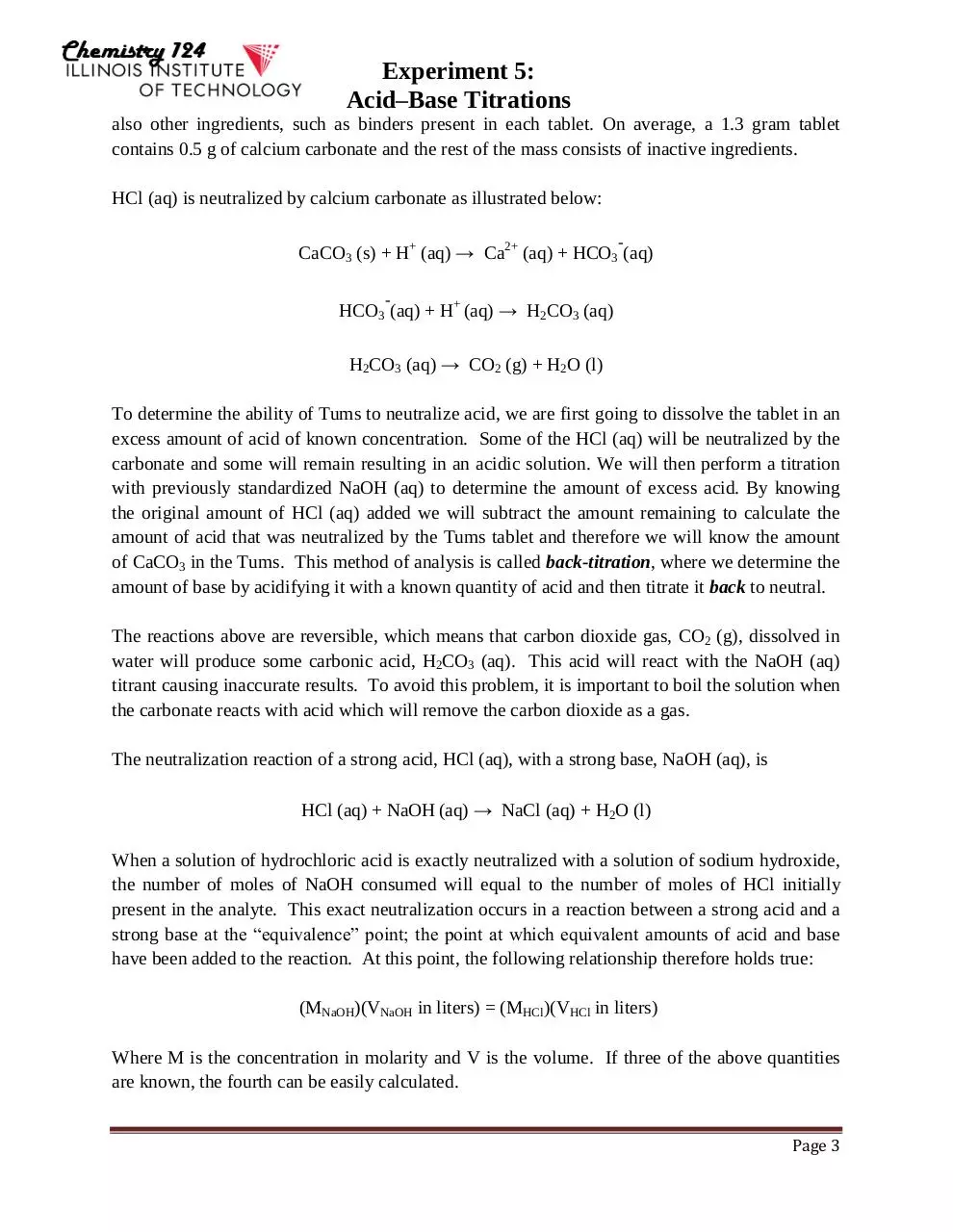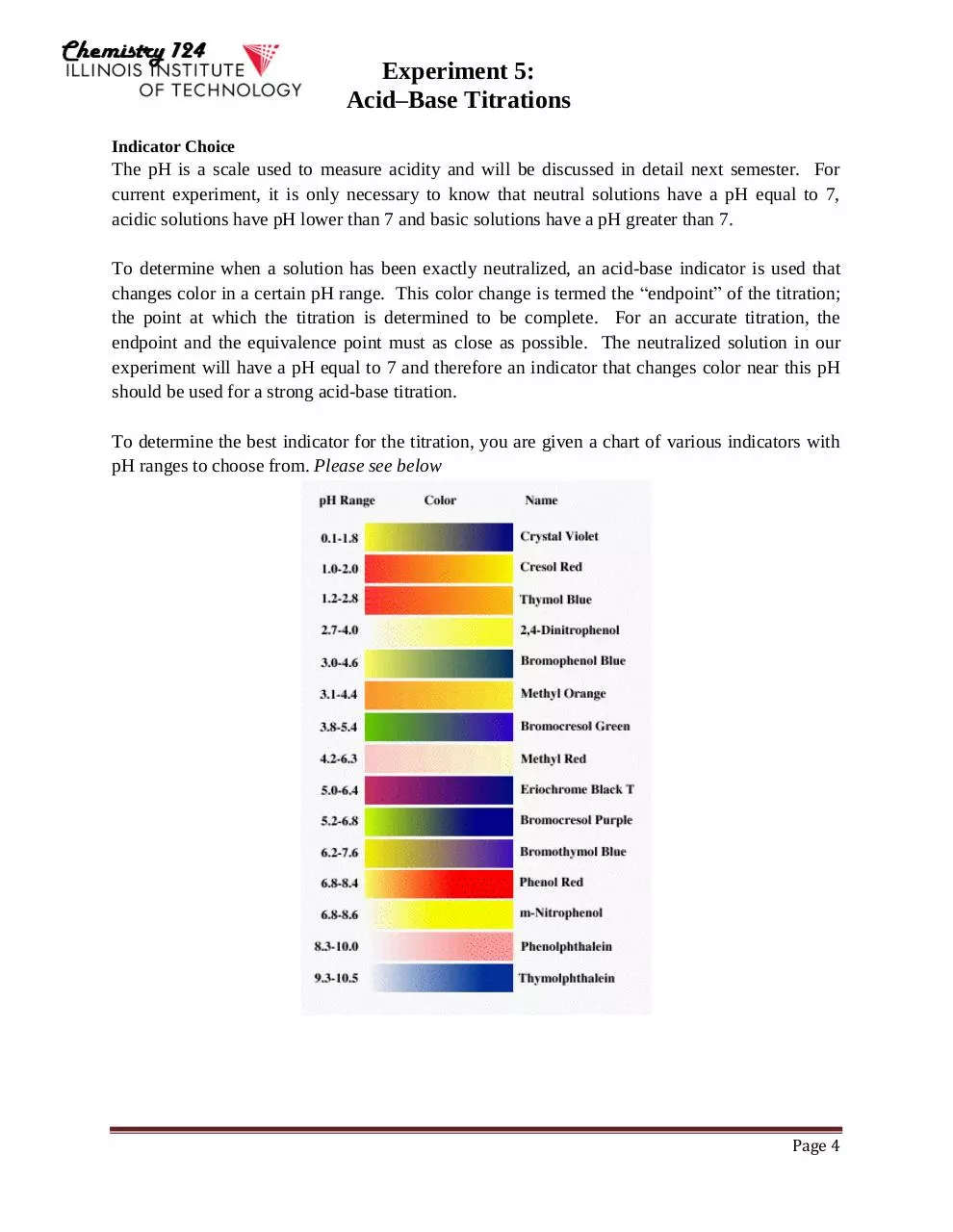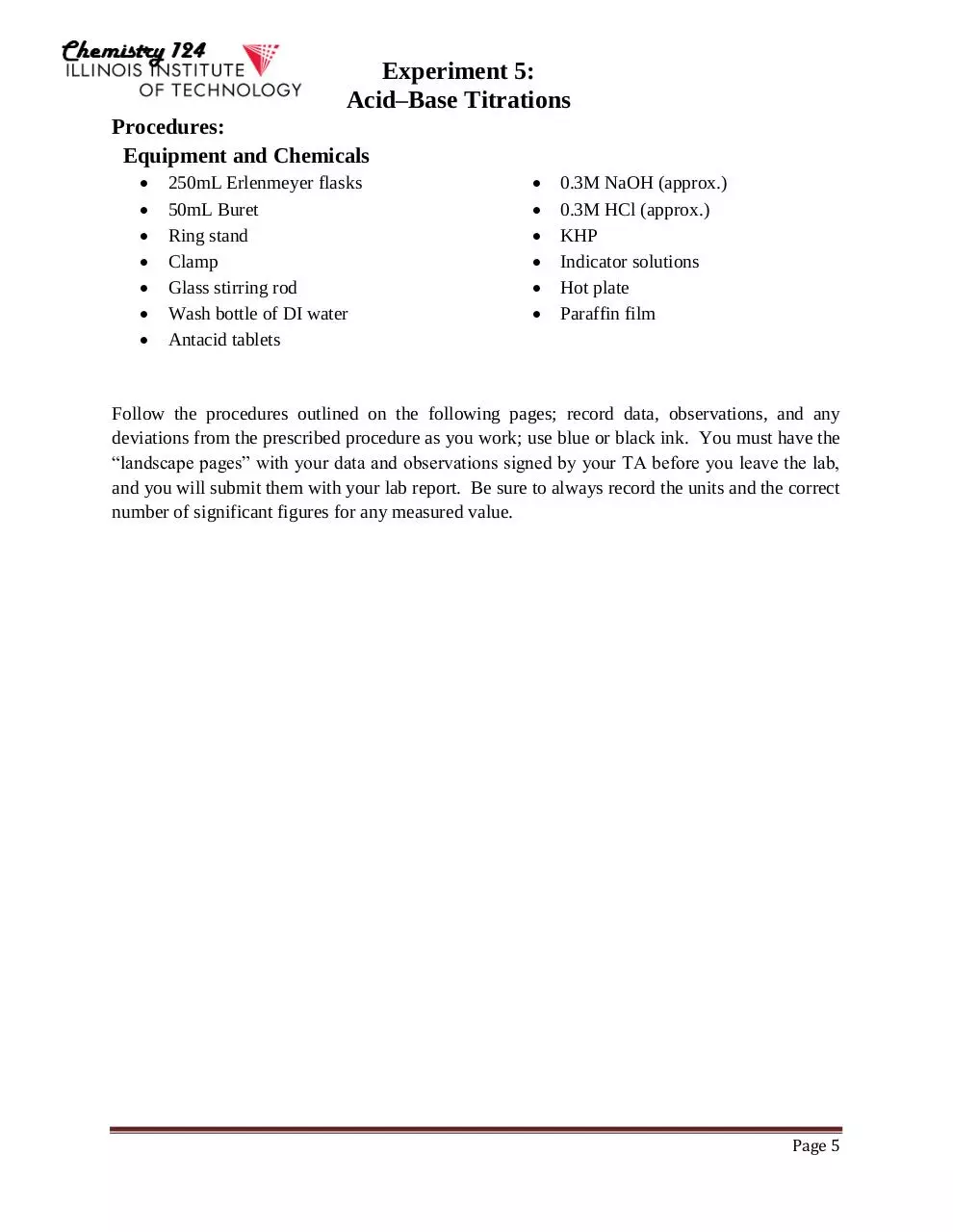Lab 5 - Titrations_Fall 2015(1).pdf (PDF, 616 KB)

### Share on social networks

#### HTML Code

Copy the following HTML code to share your document on a Website or Blog